2019-10-21 11:12:19 ljf1279 阅读数 43
• ###### Python数据分析实战-Pandas

深度学习、机器学习和数据分析必须用pandas。pandas是在python直接流行的数据处理框架。可以说，如果不会使用pandas，就谈不上会用python做数据分析。本课程会使用奥林匹克一个真实的数据作为实验数据，从初级到各种pandas的常用操作，到常用的数据可视化，让你在短的时间内掌握好pandas，轻松愉快的玩转数据分析。

2705 人正在学习 去看看 阿勒拉哈

1、dtype

``````import pandas as pd

C:/path/to/python.exe E:/data/pd.py
sys:1: DtypeWarning: Columns (3) have mixed types. Specify dtype option on import or set low_memory=False.

1、加载前设置dtype或2、设置low_memory=False

``df = pd.read_csv('path/to/file',dtype={"cat_id":int, "cat_name":object})``

``df = pd.read_csv('path/to/file',low_memory=False)``

2、内存溢出

``````# -*- coding: utf-8 -*-

# 导入必要模块
import pandas as pd
import os
from sqlalchemy import create_engine

# 初始化数据库连接，使用pymysql模块
# 切片加载数据
d = 'path'
#遍历目录
for dir in os.listdir(d):
dd = []
#遍历文件
for file in os.listdir(d.'/'.dir):
dd.append(df)
df = pd.concat(dd, ignore_index=True)
# 数据处理 略
# 写入数据库
df.to_sql('table_name', engine, index= True, if_exists='append')
``````

``````# -*- coding: utf-8 -*-

# 导入必要模块
import pandas as pd
import os
from sqlalchemy import create_engine

# 初始化数据库连接，使用pymysql模块
# 切片加载数据
d = 'path'
#遍历目录
for dir in os.listdir(d):
dd = []
#遍历文件
for file in os.listdir(d.'/'.dir):
dd.append(df)
df = pd.concat(dd, ignore_index=True)
# 数据处理 略
# 分块写数据
i = len(df.index)
size = 1000000
while i > 0 :
start = i - size
if start < 0:
start = 0
dfn = df.loc[start:i]
i=i-size
dfn.to_sql('table_name', engine, index= False, if_exists='append')
# df.to_sql('table_name', engine, index= True, if_exists='append')
``````

pandas处理大数据 相关内容

2019-06-16 13:28:17 kclax 阅读数 330
• ###### Python数据分析实战-Pandas

深度学习、机器学习和数据分析必须用pandas。pandas是在python直接流行的数据处理框架。可以说，如果不会使用pandas，就谈不上会用python做数据分析。本课程会使用奥林匹克一个真实的数据作为实验数据，从初级到各种pandas的常用操作，到常用的数据可视化，让你在短的时间内掌握好pandas，轻松愉快的玩转数据分析。

2705 人正在学习 去看看 阿勒拉哈``````在数据分析领域，最热门的莫过于Python和R语言，此前有一篇文章《别老扯什么Hadoop了，你的数据根本不够大》指出：只有在超过5TB数据量的规模下，Hadoop才是一个合理的技术选择。这次拿到近亿条日志数据，千万级数据已经是关系型数据库的查询分析瓶颈，之前使用过Hadoop对大量文本进行分类，这次决定采用Python来处理数据：

CPU：3.5 GHz Intel Core i7

Python：2.7.6
Pandas：0.15.0
IPython notebook：2.0.0

Table	Size	Desc
ServiceLogs	98,706,832 rows x 14 columns	8.77 GB	交易日志数据，

ServiceCodes	286 rows × 8 columns	20 KB	交易分类的字典表

``````

``````ipython notebook --pylab=inline
``````

Pandas提供了IO工具可以将大文件分块读取，测试了一下性能，完整加载9800万条数据也只需要263秒左右，还是相当不错了。

``````import pandas as pd
try:
except StopIteration:
print "Iteration is stopped."
``````
``````1百万条	1千万条	1亿条
ServiceLogs	1 s	17 s	263 s

``````
``````loop = True
chunkSize = 100000
chunks = []
while loop:
try:
chunks.append(chunk)
except StopIteration:
loop = False
print "Iteration is stopped."
df = pd.concat(chunks, ignore_index=True)
``````
``````下面是统计数据，Read Time是数据读取时间，Total Time是读取和Pandas进行concat操作的时间，根据数据总量来看，对5~50个DataFrame对象进行合并，性能表现比较好。

Chunk Size	Read Time (s)	Total Time (s)	Performance
100,000	224.418173	261.358521
200,000	232.076794	256.674154
1,000,000	213.128481	234.934142	√ √
2,000,000	208.410618	230.006299	√ √ √
5,000,000	209.460829	230.939319	√ √ √
10,000,000	207.082081	228.135672	√ √ √ √
20,000,000	209.628596	230.775713	√ √ √
50,000,000	222.910643	242.405967
100,000,000	263.574246	263.574246
``````Pandas提供了 DataFrame.describe 方法查看数据摘要，包括数据查看（默认共输出首尾60行数据）和行列统计。由于源数据通常包含一些空值甚至空列，会影响数据分析的时间和效率，在预览了数据摘要后，需要对这些无效数据进行处理。Pandas的非空计算速度很快，9800万数据也只需要28.7秒。得到初步信息之后，可以对表中空列进行移除操作。尝试了按列名依次计算获取非空列，和 DataFrame.dropna() 两种方式，时间分别为367.0秒和345.3秒，但检查时发现 dropna() 之后所有的行都没有了，查了Pandas手册，原来不加参数的情况下， dropna() 会移除所有包含空值的行。如果只想移除全部为空值的列，需要加上 axis 和 how 两个参数：

``````df.dropna(axis=1, how='all')
``````

``````df['Name'] = df['Name'].astype(np.datetime64)
``````

``````df.groupby(['NO','TIME','SVID']).count() # 分组
fullData = pd.merge(df, trancodeData)[['NO','SVID','TIME','CLASS','TYPE']] # 连接
actions = fullData.pivot_table('SVID', columns='TYPE', aggfunc='count') # 透视表
````````````total_actions = fullData.pivot_table('SVID', index='TIME', columns='TYPE', aggfunc='count')
total_actions.plot(subplots=False, figsize=(18,6), kind='area')
````````````tranData = fullData[fullData['Type'] == 'Transaction']
``````

pandas处理大数据 相关内容

2019-02-11 17:21:57 yiluohan0307 阅读数 827
• ###### Python数据分析实战-Pandas

深度学习、机器学习和数据分析必须用pandas。pandas是在python直接流行的数据处理框架。可以说，如果不会使用pandas，就谈不上会用python做数据分析。本课程会使用奥林匹克一个真实的数据作为实验数据，从初级到各种pandas的常用操作，到常用的数据可视化，让你在短的时间内掌握好pandas，轻松愉快的玩转数据分析。

2705 人正在学习 去看看 阿勒拉哈

# python pandas处理大数据节省内存的方法

• 数值类型的列进行降级处理
• 字符串类型的列转化为类别类型（category）
• 字符串类型的列的类别数超过总行数的一半时，建议使用object类型
``````'''

'''
def reduce_mem_usage(df, verbose=True):
numerics = ['int16', 'int32', 'int64', 'float16', 'float32', 'float64', 'object']
start_mem = df.memory_usage().sum() / 1024**2
if verbose:print "Memory usage of the dataframe before converted is :", start_mem, "MB"
# print dataset.isnull().any()
for col in df.columns:
col_type = df[col].dtypes
if col_type in numerics:
c_min = df[col].min()
c_max = df[col].max()
if str(col_type)[:3] == 'int':
if c_min > np.iinfo(np.int8).min and c_max < np.iinfo(np.int8).max:
df[col] = df[col].astype(np.int8)
elif c_min > np.iinfo(np.int16).min and c_max < np.iinfo(np.int16).max:
df[col] = df[col].astype(np.int16)
elif c_min > np.iinfo(np.int32).min and c_max < np.iinfo(np.int32).max:
df[col] = df[col].astype(np.int32)
elif c_min > np.iinfo(np.int64).min and c_max < np.iinfo(np.int64).max:
df[col] = df[col].astype(np.int64)
elif str(col_type)[:5] == 'float':
if c_min > np.finfo(np.float16).min and c_max < np.finfo(np.float16).max:
df[col] = df[col].astype(np.float16)
elif c_min > np.finfo(np.float32).min and c_max < np.finfo(np.float32).max:
df[col] = df[col].astype(np.float32)
else:
df[col] = df[col].astype(np.float64)
else:
num_unique_values = len(df[col].unique())
num_total_values = len(df[col])
rate = num_unique_values/num_total_values
#rate = df[col].value_counts(normalize=True, dropna=False).values
if rate <0.5:
df[col] = df[col].astype('category')
end_mem = df.memory_usage().sum() / 1024**2
if verbose:print "Memory usage of the dataframe after converted is :", end_mem, "MB"
if verbose: print('Mem. usage decreased to {:5.2f} Mb ({:.1f}% reduction)'.format(end_mem, 100 * (start_mem - end_mem) / start_mem))
return df, ditc(df.types)
``````

## 参考

https://blog.csdn.net/weiyongle1996/article/details/78498603

https://blog.csdn.net/qq_34739497/article/details/79600479

pandas处理大数据 相关内容

2020-02-07 10:08:54 haoxun04 阅读数 450
• ###### Python数据分析实战-Pandas

深度学习、机器学习和数据分析必须用pandas。pandas是在python直接流行的数据处理框架。可以说，如果不会使用pandas，就谈不上会用python做数据分析。本课程会使用奥林匹克一个真实的数据作为实验数据，从初级到各种pandas的常用操作，到常用的数据可视化，让你在短的时间内掌握好pandas，轻松愉快的玩转数据分析。

2705 人正在学习 去看看 阿勒拉哈

``````硬件环境
CPU：3.5 GHz Intel Core i7
内存：32 GB HDDR 3 1600 MHz
硬盘：3 TB Fusion Drive

Python：2.7.6
Pandas：0.15.0
IPython notebook：2.0.0
``````ipython notebook --pylab=inline

Pandas提供了IO工具可以将大文件分块读取，测试了一下性能，完整加载9800万条数据也只需要263秒左右，还是相当不错了。

import pandas as pd
try:
except StopIteration:
print “Iteration is stopped.”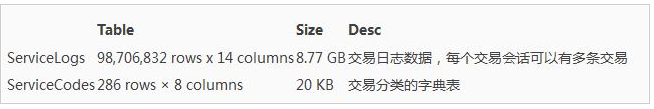``````    loop = True
chunkSize = 100000
chunks = []
while loop:
try:
chunks.append(chunk)
except StopIteration:
loop = False
print "Iteration is stopped."
df = pd.concat(chunks, ignore_index=True)
``````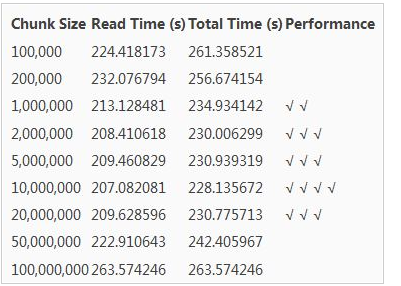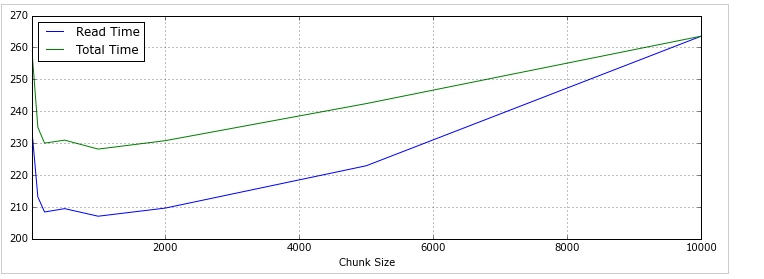Pandas提供了 DataFrame.describe 方法查看数据摘要，包括数据查看（默认共输出首尾60行数据）和行列统计。由于源数据通常包含一些空值甚至空列，会影响数据分析的时间和效率，在预览了数据摘要后，需要对这些无效数据进行处理。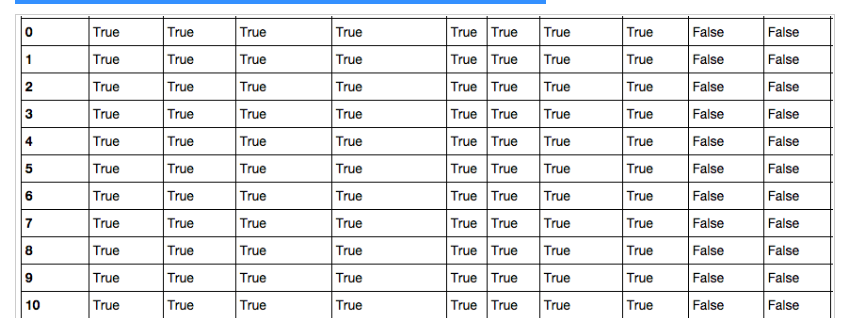Pandas的非空计算速度很快，9800万数据也只需要28.7秒。得到初步信息之后，可以对表中空列进行移除操作。尝试了按列名依次计算获取非空列，和 DataFrame.dropna() 两种方式，时间分别为367.0秒和345.3秒，但检查时发现 dropna() 之后所有的行都没有了，查了Pandas手册，原来不加参数的情况下， dropna() 会移除所有包含空值的行。如果只想移除全部为空值的列，需要加上 axis 和 how 两个参数：

``````df.dropna(axis=1, how='all')
``````

、爬虫、django、数据挖掘等【PDF，实战源码】，零基础到项目实战的资料都有整理。送给每一位

python的小伙伴！每天都有大牛定时讲解Python技术，分享一些学习的方法和需要注意的小细节，点击

``````df['Name'] = df['Name'].astype(np.datetime64)
``````

``````df.groupby(['NO','TIME','SVID']).count() # 分组
fullData = pd.merge(df, trancodeData)[['NO','SVID','TIME','CLASS','TYPE']] # 连接
actions = fullData.pivot_table('SVID', columns='TYPE', aggfunc='count') # 透视表
``````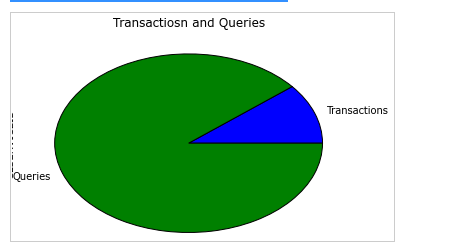``````total_actions = fullData.pivot_table('SVID', index='TIME', columns='TYPE', aggfunc='count')
total_actions.plot(subplots=False, figsize=(18,6), kind='area')
``````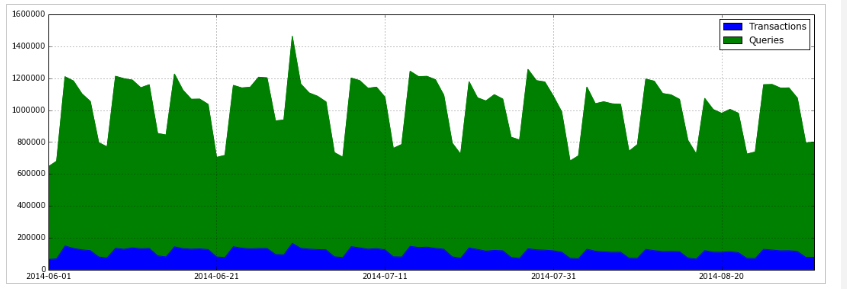``````tranData = fullData[fullData['Type'] == 'Transaction']
``````

pandas处理大数据 相关内容

2017-08-29 21:54:51 wally21st 阅读数 5525
• ###### Python数据分析实战-Pandas

深度学习、机器学习和数据分析必须用pandas。pandas是在python直接流行的数据处理框架。可以说，如果不会使用pandas，就谈不上会用python做数据分析。本课程会使用奥林匹克一个真实的数据作为实验数据，从初级到各种pandas的常用操作，到常用的数据可视化，让你在短的时间内掌握好pandas，轻松愉快的玩转数据分析。

2705 人正在学习 去看看 阿勒拉哈

## 处理棒球比赛记录数据

``````import pandas as pd

date number_of_game day_of_week v_name v_league v_game_number h_name
0 18710504 0 Thu CL1 na 1 FW1
1 18710505 0 Fri BS1 na 1 WS3
2 18710506 0 Sat CL1 na 2 RC1
3 18710508 0 Mon CL1 na 3 CH1
4 18710509 0 Tue BS1 na 2 TRO

• `date` - 比赛日期
• `v_name` - 客队名
• `v_league` - 客队联赛
• `h_name` - 主队名
• `h_league` - 主队联赛
• `v_score` - 客队得分
• `h_score` - 主队得分
• `v_line_score` - 客队线得分, 如010000(10)00.
• `h_line_score`- 主队线得分, 如010000(10)0X.
• `park_id` - 主办场地的ID
• `attendance`- 比赛出席人数

``gl.info(memory_usage='deep')``
``````<class 'pandas.core.frame.DataFrame'>
RangeIndex: 171907 entries, 0 to 171906
Columns: 161 entries, date to acquisition_info
dtypes: float64(77), int64(6), object(78)
memory usage: 861.6 MB
``````

## Dataframe对象的内部表示``````for dtype in ['float','int','object']:
selected_dtype = gl.select_dtypes(include=[dtype])
mean_usage_b = selected_dtype.memory_usage(deep=True).mean()
mean_usage_mb = mean_usage_b / 1024 ** 2
print("Average memory usage for {} columns: {:03.2f} MB".format(dtype,mean_usage_mb))``````
``````Average memory usage for float columns: 1.29 MB
Average memory usage for int columns: 1.12 MB
Average memory usage for object columns: 9.53 MB
``````

## 理解子类型(Subtypes)

pandas中的许多数据类型具有多个子类型，它们可以使用较少的字节去表示不同数据，比如，`float`型就有`float16``float32``float64`这些子类型。这些类型名称的数字部分表明了这种类型使用了多少比特来表示数据，比如刚才列出的子类型分别使用了2、4、8个字节。下面这张表列出了pandas中常用类型的子类型：

memory usage float int uint datetime bool object
1 bytes int8 uint8 bool
2 bytes float16 int16 uint16
4 bytes float32 int32 uint32
8 bytes float64 int64 uint64 datetime64
variable object

``````import numpy as np
int_types = ["uint8", "int8", "int16"]
for it in int_types:
print(np.iinfo(it))``````
``````Machine parameters for uint8
-----------------------------------------------------
min = 0
max = 255
-----------------------------------------------------

Machine parameters for int8
-----------------------------------------------------
min = -128
max = 127
-----------------------------------------------------

Machine parameters for int16
-----------------------------------------------------
min = -32768
max = 32767
-----------------------------------------------------
``````

## 用子类型优化数值型列

``````# We're going to be calculating memory usage a lot,
# so we'll create a function to save us some time!

def mem_usage(pandas_obj):
if isinstance(pandas_obj,pd.DataFrame):
usage_b = pandas_obj.memory_usage(deep=True).sum()
else: # we assume if not a df it's a series
usage_b = pandas_obj.memory_usage(deep=True)
usage_mb = usage_b / 1024 ** 2 # convert bytes to megabytes
return "{:03.2f} MB".format(usage_mb)

gl_int = gl.select_dtypes(include=['int'])
converted_int = gl_int.apply(pd.to_numeric,downcast='unsigned')

print(mem_usage(gl_int))
print(mem_usage(converted_int))

compare_ints = pd.concat([gl_int.dtypes,converted_int.dtypes],axis=1)
compare_ints.columns = ['before','after']
compare_ints.apply(pd.Series.value_counts)``````
``````7.87 MB
1.48 MB
``````
before after
uint8 NaN 5.0
uint32 NaN 1.0
int64 6.0 NaN

``````gl_float = gl.select_dtypes(include=['float'])
converted_float = gl_float.apply(pd.to_numeric,downcast='float')

print(mem_usage(gl_float))
print(mem_usage(converted_float))

compare_floats = pd.concat([gl_float.dtypes,converted_float.dtypes],axis=1)
compare_floats.columns = ['before','after']
compare_floats.apply(pd.Series.value_counts)``````
``````100.99 MB
50.49 MB
``````
before after
float32 NaN 77.0
float64 77.0 NaN

``````optimized_gl = gl.copy()

optimized_gl[converted_int.columns] = converted_int
optimized_gl[converted_float.columns] = converted_float

print(mem_usage(gl))
print(mem_usage(optimized_gl))``````
``````861.57 MB
804.69 MB
``````

## 对比数值与字符的存储

`object`类型用来表示用到了Python字符串对象的值，有一部分原因是Numpy缺少对缺失字符串值的支持。因为Python是一种高层、解析型语言，它没有提供很好的对内存中数据如何存储的细粒度控制。``````from sys import getsizeof

s1 = 'working out'
s2 = 'memory usage for'
s3 = 'strings in python is fun!'
s4 = 'strings in python is fun!'

for s in [s1, s2, s3, s4]:
print(getsizeof(s))``````
``````60
65
74
74
``````
``````obj_series = pd.Series(['working out',
'memory usage for',
'strings in python is fun!',
'strings in python is fun!'])
obj_series.apply(getsizeof)``````
``````0    60
1    65
2    74
3    74
dtype: int64
``````

## 用类别（categoricals）类型优化object类型

Pandas在0.15版本中引入类别类型。`category`类型在底层使用整型数值来表示该列的值，而不是用原值。Pandas用一个字典来构建这些整型数据到原数据的映射关系。当一列只包含有限种值时，这种设计是很不错的。当我们把一列转换成`category`类型时，pandas会用一种最省空间的`int`子类型去表示这一列中所有的唯一值。``````gl_obj = gl.select_dtypes(include=['object']).copy()
gl_obj.describe()``````
day_of_week v_name v_league h_name h_league day_night
count 171907 171907 171907 171907 171907 140150
unique 7 148 7 148 7 2
top Sat CHN NL CHN NL D
freq 28891 8870 88866 9024 88867 82724

``````dow = gl_obj.day_of_week

dow_cat = dow.astype('category')
``````0    Thu
1    Fri
2    Sat
3    Mon
4    Tue
Name: day_of_week, dtype: object
0    Thu
1    Fri
2    Sat
3    Mon
4    Tue
Name: day_of_week, dtype: category
Categories (7, object): [Fri, Mon, Sat, Sun, Thu, Tue, Wed]
``````

``dow_cat.head().cat.codes``
``````0    4
1    0
2    2
3    1
4    5
dtype: int8
``````

``````print(mem_usage(dow))
print(mem_usage(dow_cat))``````
``````9.84 MB
0.16 MB
``````

``````converted_obj = pd.DataFrame()

for col in gl_obj.columns:
num_unique_values = len(gl_obj[col].unique())
num_total_values = len(gl_obj[col])
if num_unique_values / num_total_values < 0.5:
converted_obj.loc[:,col] = gl_obj[col].astype('category')
else:
converted_obj.loc[:,col] = gl_obj[col]``````

``````print(mem_usage(gl_obj))
print(mem_usage(converted_obj))

compare_obj = pd.concat([gl_obj.dtypes,converted_obj.dtypes],axis=1)
compare_obj.columns = ['before','after']
compare_obj.apply(pd.Series.value_counts)``````
``````752.72 MB
51.67 MB
``````
before after
object 78.0 NaN
category NaN 78.0

``````optimized_gl[converted_obj.columns] = converted_obj

mem_usage(optimized_gl)``````
``````'103.64 MB'
``````

``````date = optimized_gl.date
print(mem_usage(date))
``````0.66 MB
0    18710504
1    18710505
2    18710506
3    18710508
4    18710509
Name: date, dtype: uint32
``````

``````optimized_gl['date'] = pd.to_datetime(date,format='%Y%m%d')

print(mem_usage(optimized_gl))
``````104.29 MB
0   1871-05-04
1   1871-05-05
2   1871-05-06
3   1871-05-08
4   1871-05-09
Name: date, dtype: datetime64[ns]
``````

## 在数据读入的时候设定数据类型

``````dtypes = optimized_gl.drop('date',axis=1).dtypes

dtypes_col = dtypes.index
dtypes_type = [i.name for i in dtypes.values]

column_types = dict(zip(dtypes_col, dtypes_type))

# rather than print all 161 items, we'll
# sample 10 key/value pairs from the dict
# and print it nicely using prettyprint

preview = first2pairs = {key:value for key,value in list(column_types.items())[:10]}
import pprint
pp = pp = pprint.PrettyPrinter(indent=4)
pp.pprint(preview)``````
``````{   'acquisition_info': 'category',
'h_caught_stealing': 'float32',
'h_player_1_name': 'category',
'h_player_9_name': 'category',
'v_assists': 'float32',
'v_first_catcher_interference': 'float32',
'v_grounded_into_double': 'float32',
'v_player_1_id': 'category',
'v_player_3_id': 'category',
'v_player_5_id': 'category'}
``````

``````read_and_optimized = pd.read_csv('game_logs.csv',dtype=column_types,parse_dates=['date'],infer_datetime_format=True)

``````104.28 MB
``````
date number_of_game day_of_week v_name v_league v_game_number h_name
0 1871-05-04 0 Thu CL1 na 1 FW1
1 1871-05-05 0 Fri BS1 na 1 WS3
2 1871-05-06 0 Sat CL1 na 2 RC1
3 1871-05-08 0 Mon CL1 na 3 CH1
4 1871-05-09 0 Tue BS1 na 2 TRO

## 分析棒球比赛

``````optimized_gl['year'] = optimized_gl.date.dt.year
games_per_day = optimized_gl.pivot_table(index='year',columns='day_of_week',values='date',aggfunc=len)
games_per_day = games_per_day.divide(games_per_day.sum(axis=1),axis=0)

ax = games_per_day.plot(kind='area',stacked='true')
ax.legend(loc='upper right')
ax.set_ylim(0,1)
plt.show()````````````game_lengths = optimized_gl.pivot_table(index='year', values='length_minutes')
game_lengths.reset_index().plot.scatter('year','length_minutes')
plt.show()``````## 总结

• 将数值型列降级到更高效的类型
• 将字符串列转换为类别类型

pandas处理大数据 相关内容